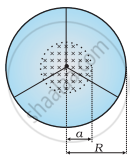# A line charge λ per unit length is lodged uniformly onto the rim of a wheel of mass M and radius R. - Physics

Numerical

A line charge λ per unit length is lodged uniformly onto the rim of a wheel of mass M and radius R. The wheel has light non-conducting spokes and is free to rotate without friction about its axis (Figure). A uniform magnetic field extends over a circular region within the rim. It is given by,

B = − B0 k (r ≤ a; a < R)

= 0 (otherwise)

What is the angular velocity of the wheel after the field is suddenly switched off?#### Solution

Line charge per unit length = λ = "Total charge"/"Length" = "Q"/(2π"r")

Where,

r = Distance of the point within the wheel

Mass of the wheel = M

Radius of the wheel = R

Magnetic field, vec"B" = -"B"_0hat"k"

At distance r,themagnetic force is balanced by the centripetal force i.e.,

"BQv" = ("mv"^2)/"r"

Where

v = linear velocity of the wheel

∴ "B"2pi"r"lambda = ("Mv")/"r"

"v" = ("B"2pilambda"r"^2)/"M"

∴ Angular velocity, omega = "v"/"r" = ("B"2pilambda"r"^2)/("MR")

For r ≤ and a < R, we get:

omega = -(2pi"B""aa"^2lambda)/("MR")hat"k"

Concept: Electromagnetic Induction
Is there an error in this question or solution?

#### APPEARS IN

NCERT Physics Part 1 and 2 Class 12
Chapter 6 Electromagnetic Induction
Exercise | Q 6.17 | Page 232
NCERT Class 12 Physics Textbook
Chapter 6 Electromagnetic Induction
Exercise | Q 17 | Page 232

Share